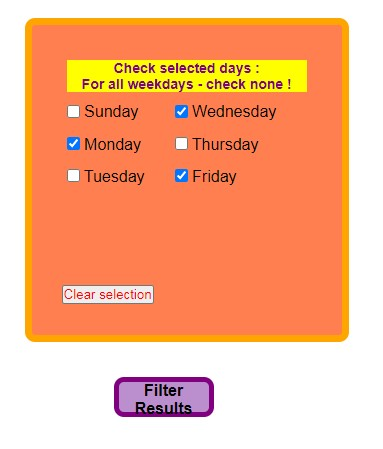# Array to string conversion problem

Hello ,

I have a database of trades

I want to filter them by weekdays

This is the formI want to be able to filter trades made on momdays, Mondays and Wednesdays, or any combination of weekdays.

The \$days variable which gets the days selection is an array.
var_dump(\$days) gives

``````array (size=3)*
0 => string '0' *(length=1)*
1 => string '2' *(length=1)*
2 => string '4' *(length=1)*

I
``````

n order to use the filter criteria until I chagge it I store it in a table

SQL tables can’t store arrays so I use implode to cange the array into a string
`\$weekdays = implode("",\$days);`

When I want to use it I use explode to turn the string to an array

``````\$filter_criteria = get_user_filter_criteria(\$user_id);

foreach(\$filter_criteria as \$xrow =>\$xval)
{
\$start_date = \$xval['start_date'];
\$end_date = \$xval['end_date'];
//\$weekdays = \$xval['weekdays'];
\$hour_start = \$xval['hour_start'];
\$hour_end = \$xval['hour_end'];
}
echo \$xval['weekdays'];
Insert days selected into \$days array
\$days = explode(\$weekdays);
``````

(All other filter criteria work well)
echo \$xval[‘weekdays’]; gives 024

var_dump(\$days); gives
array (size=1) 0 => string ‘024’ (length=3)

But I get a notice array to string conversion in

``````\$sql = "SELECT item, SUM(profit) AS profit, o_time
FROM data
WHERE c_time BETWEEN :start_date AND :end_date
AND TIME(`o_time`) BETWEEN :hour_start AND :hour_end
AND WEEKDAY(`o_time`) IN (\$days)
GROUP BY item ";
``````

in `AND WEEKDAY(`o_time`) IN (\$days)`

why does it happen ? how do I fix it ?

well no, thats not what you said before.

will not give you this result. It will generate an error because explode expects 2 parameters, and you’re only giving it one.

You’ve confused `explode` and `str_split`.

m_hutley, Thanks for the quick response. I did mixed it up. Thus is because I tried every possible sulution I found on the web before I posted here.

I ipploded selected days

``````\$weekdays  = implode(" ",\$days);
\$days = \$_POST['weekday'];
``````

When I extracted it from the database I exploded it

``````echo \$xval['weekdays'];
// Insert days selected into \$days array
\$days = explode(" ",\$xval['weekdays']);

var_dump(\$days);
``````

I got

``````0 2 4

array (size=3)
0 => string  '0' *(length=1)
1 => string  '2' *(length=1)
2 => string  '4' *(length=1)*
``````

and
Notice: Array to string conversion in C:\wamp\www\trade_analyzer_filter_NEWֹTABLESֹ\fetch.php on line 617

This is line 617
`AND WEEKDAY(`o_time`) IN (\$days)`

I also tried `str_split` . whem I imploded selected days with no space between them
I tried echo json encode()